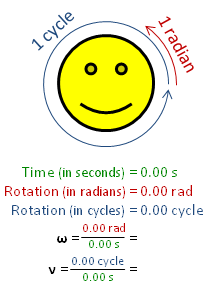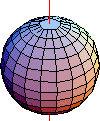# Angular frequencyAngular frequency ω (in radians per second), is larger than frequency ν (in cycles per second, also called Hz), by a factor of 2π. This figure uses the symbol ν, rather than f to denote frequency.A sphere rotating around an axis. Points farther from the axis move faster, satisfying ω=v/r.

In physics, angular frequency ω (also referred to by the terms angular speed, radial frequency, circular frequency, orbital frequency, radian frequency, and pulsatance) is a scalar measure of rotation rate. It refers to the angular displacement per unit time (e.g., in rotation) or the rate of change of the phase of a sinusoidal waveform (e.g., in oscillations and waves), or as the rate of change of the argument of the sine function.

Angular frequency (or angular speed) is the magnitude of the vector quantity angular velocity. The term angular frequency vectoris sometimes used as a synonym for the vector quantity angular velocity.

One revolution is equal to 2π radians, hencewhere:

ω is the angular frequency or angular speed (measured in radians per second),
T is the period (measured in seconds),
f is the ordinary frequency (measured in hertz) (sometimes symbolised with ν).

## Units

In SI units, angular frequency is normally presented in radians per second, even when it does not express a rotational value. From the perspective of dimensional analysis, the unit hertz (Hz) is also correct, but in practice it is only used for ordinary frequency f, and almost never for ω. This convention helps avoid confusion.

In digital signal processing, the angular frequency may be normalized by the sampling rate, yielding the normalized frequency.

## Circular motion

Main article: Circular motion

In a rotating or orbiting object, there is a relation between distance from the axis, tangential speed, and the angular frequency of the rotation:### Oscillations of a spring

An object attached to a spring will oscillate. Assuming that the spring is ideal and massless with no damping then the motion will be simple and harmonic with an angular frequency given by:where

k is the spring constant
m is the mass of the object.

ω is referred to as the natural frequency (which can sometimes be denoted as ω0).

As the object oscillates, its acceleration can be calculated bywhere x is displacement from an equilibrium position.

Using 'ordinary' revolutions-per-second frequency, this equation would be### LC circuits

The resonant angular frequency in an LC circuit equals the square root of the inverse of the product of the capacitance (C measured in farads) and the inductance of the circuit (L in henrys).## References and notes

1. Cummings, Karen; Halliday, David (Second Reprint: 2007). Understanding physics. New Delhi: John Wiley & Sons Inc., authorized reprint to Wiley - India. pp. 449, 484, 485, 487. ISBN 978-81-265-0882-2. Check date values in: |date= (help)(UP1)
2. Holzner, Steven (2006). Physics for Dummies. Hoboken, New Jersey: Wiley Publishing Inc. p. 201. ISBN 978-0-7645-5433-9.
3. Lerner, Lawrence S. (1996-01-01). Physics for scientists and engineers. p. 145. ISBN 978-0-86720-479-7.
4. Serway,, Raymond A.; Jewett, John W. (2006). Principles of physics - 4th Edition. Belmont, CA.: Brooks / Cole - Thomson Learning. pp. 375, 376, 385, 397. ISBN 978-0-534-46479-0.
5. Nahvi, Mahmood; Edminister, Joseph (2003). Schaum's outline of theory and problems of electric circuits. McGraw - Hill Companies (McGraw - Hill Professional). pp. 214, 216. ISBN 0-07-139307-2.(LC1)